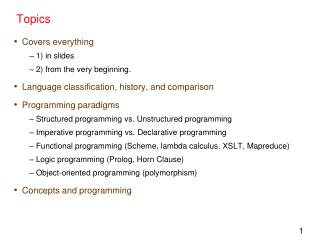DownloadDownload PresentationTopics

# Topics

Download Presentation## Topics

- - - - - - - - - - - - - - - - - - - - - - - - - - - E N D - - - - - - - - - - - - - - - - - - - - - - - - - - -
##### Presentation Transcript

1. Topics • Covers everything • 1) in slides • 2) from the very beginning. • Language classification, history, and comparison • Programming paradigms • Structured programming vs. Unstructured programming • Imperative programming vs. Declarative programming • Functional programming (Scheme, lambda calculus, XSLT, Mapreduce) • Logic programming (Prolog, Horn Clause) • Object-oriented programming (polymorphism) • Concepts and programming

2. Types of questions • Multiple choices; true/false; filling in blanks. • Running results of programs; • Modify programs; • Short explanations; • Others.

3. BNF was first used to describe the syntax of which language: A. C B. Fortran C. Algol D. COBOL E. LISP F. None of the above

4. Which of the following language is not a declarative language? A. Java B. Prolog C. SQL D. Scheme E. None of the above.

5. Which of the following is the closest to the theoretical foundation of Prolog language? • BNF; • Horn clause; • Lambda calculus; • First order logic; • None of the above.

6. Which one of the following is not a type of polymorphism • coercion • overloading • overriding • generics • All of them are types of polymorphism

7. Given the expression ((x. x)x.x)a in lambda calculus. Derive the expression as far as possible using β-reduction. The final result will be: • (x.x)a • x.x • xa • a • none of the above

8. Given the expression ((x.x)(x.xxx))a in lambda calculus. Derive the expression as far as possible using β-reduction. The final result will be: • xx • x.x • xa • a • aa • none above

9. ( x y . x y) ( x . x y) ( a b . a b) ( x z . x z) ( x . x y) ( a b . a b)  conversion ( z . ( x . x y) z) ( a b . a b)  reduction ( x . x y) ( a b . a b)  reduction ( a b . a b) y  reduction • ( b . y b)  reduction • y  reduction

10. What is the value of (map (lambda (x) (* 2 x)) (1 2 3)) ? • a run-time error • ( ) (the empty list) • (3 4 5) • (2 4 6) • 12 • none of the above • What is the value of (map (lambda (x) (* 2 x)) '(1 2 3)) ? • What is the value of (eval (map (lambda (x) (* 2 x)) '(1 2 3)))?

11. Given a Prolog rule A:-B,C. It is equivalent to

12. General form of a rule (Horn Clause) •     A :- B1, B2, ...., Bn. • meaning A is true if B1 and B2 and ... Bn are all true • Clause: a disjunction of literals • Horn clause: if it contains at most one positive literal.

13. True or False? • XPath is a language that is not in XML format. • XPath is part of XSLT. • Both Scheme and Prolog are declarative languages. • In Prolog, changing the order of subgoals will not lead to different results.

14. edge(a,b). edge(b,c). edge(c,d). edge(d,e). edge(b,e). edge(d,f). path(X,Y):-edge(X,Z), path(Z,Y). path(X,X).

15. The expressive power of Prolog is stronger than first order logic. • Prolog is mainly for AI applications.

16. Write the result of running the following Scheme programs. (define (f lst) (if (null? lst) lst (append (f (cdr lst)) (list (car lst))))) (f '(1 2 3)) % reverse? (define (g lst n) ; assumes lst is non-empty and n >= 0 (if (= n 0) (car lst) (g (cdr lst) (- n 1)))) (g '(1 2 3 4) 2) %n-th element

17. rainy(seattle). rainy(rochester). cold(rochester). snowy(X):-rainy(X), cold(X). p([ ],[ ]). p([H|T],L) :- p(T,Z), append(Z,[H],L). likes(john, mary). likes(dwight, X). likes(john, X):-likes(mary, X). likes(mary, sue). Write the answer(s) of the following queries. If there are more than one answer, write all of them. | ?- snowy(X). | ?- p([1, 2, 3], X). | ?- likes(X, mary).

18. (define f (lambda (x) (lambda (y) (+ x y)))) (define (g x) ((f x) 3)) (define h (lambda (x) (lambda (y) (y x)))) (define (p f) ((h 2) f)) • a). What is the return value of (g 2)? 5 • b). What is the return value of (p +)? 2

19. Assuming that the following definitions are executed in this order: (define b ‘(3 14 27)) (define c (cons (car (cdr b)) (list ‘a ‘b ‘c))) • What is the result of typing the following into the Scheme interpreter: • c • (14 a b c) • (car (cdr (cdr c))) • b

20. Given the following XML document as an input for XSLT programs, answer the following questions: <source> <employee> <firstName>Joe</firstName> <surName>Smith</surName> </employee> <employee> <firstName>Andrew</firstName> <surName>Wang</surName> <supervisor> <employee> <firstName>Steve</firstName> <surName>Miller</surName> </employee> </supervisor> </employee> </source> Write the output of the following XSLT program. You don’t need to write the exact spaces and carriage returns. <xsl:stylesheet xmlns:xsl="http://www.w3.org/1999/XSL/Transform" version="1.0"> <xsl:template match="employee/supervisor/employee"> first name is <xsl:value-of select="firstName"/> </xsl:template> </xsl:stylesheet>